Next: Orbits of Galaxies Up: Optimization Previous: The Newton-Raphson Method

## Secant Method

Comparing the iterative formula of the Steepest Descent (4.5) with (4.22) shows that the Steepest Decent method is identical to the Newton-Raphson when,being the unit matrix. This is the basis for the Secant method, also known as the Variable Metric method.As seen in the previous subsection, the Hessian matrix needed in the Newton-Raphson method can be both laborious to calculate and invert for systems with a large number of dimensions. The idea of the Secant method is not to use the Hessian matrix directly, but rather start of the procedure with an approximation to the matrix, which, as one gets closer to the solution, gradually approaches the Hessian.

The earliest Secant methods were formulated in terms of constructing a sequence of matriceswhich builds up the inverse Hessian; that is,Even better if the limit is achieved after n iterations instead of infinitely many iterations. The iterative formula is then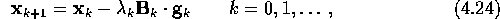where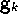is the gradient. Later, methods for constructing the ordinary Hessian were presented. The inverse Hessian approximation might seem to offer an advantage in terms of the number of arithmetic operation required to perform an iteration, since one needs to inverse the matrix for the Hessian update. This seeming defect was eliminated by, instead of updating the Hessian, the Cholesky factorsof the Hessian were modified at each iteration GMW. The number of arithmetic operations is then the same for the two methods. Both methods are being used today, the choice being a matter of convenience and taste. The inverse Hessian update will be used in this thesis, and therefore only this method will be presented in the following. For details on the Hessian update, see GMW,DS.

For a n-dimensional quadratic function, the inverse Hessian will be achieved after at most n iterations, and the solution will then be located, from what we saw in Subsection 4.3.3, in only one iteration. Hence, the solution will be arrived at after at most n iterations. In other words, this method resembles the method of Steepest Descent near the starting point of the procedure, while near the optimal point, it metamorphose into the Newton-Raphson method.

The Secant method is quite similar to the Conjugate Gradients, in some senses. They both converge after n iterations for an n-dimensional quadratic function, they both accumulate information from successive iterations, and they both require the calculation of only the first derivative. (The latter makes the Secant method part of category (2) from page.) The main difference between the two methods is the way they store and update the information; the Secant method requires anmatrix while the Conjugate Gradients method only needs an n-dimensional vector. Usually, the storage of the matrix in the Secant method is no disadvantage, at least not for a moderate number of variables.

So how are thebeing updated at each iteration? There are two main schemes GMW, namely Davidon-Fletcher-Powell (DFP) and Broyden-Fletcher-Goldfarb-Shanno (BFGS). Let us first consider the DFP algorithm. At a point, the approximated inverse Hessian at the subsequent point is given by Press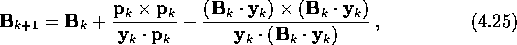where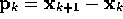and,being the gradient. This expression is constructed by forcing the-s to be mutually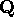-conjugate. Even though the deduction of equation (4.25) is in regard of a quadratic function, it basically applies for all kinds of equations/systems. Another property of (4.25) is that if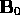is positive definite, it will remain so for each k; i.e.is a sequence of positive definite matrices. For proofs and a detailed deduction of (4.25), see Walsh.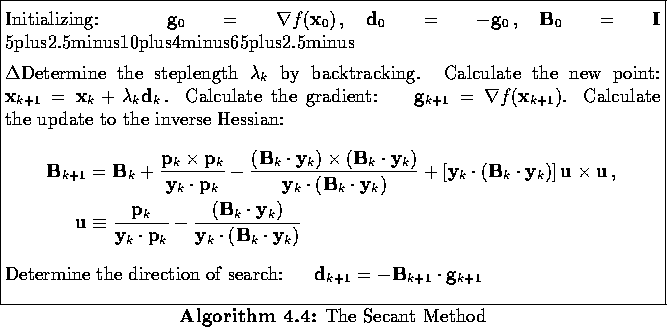The BFGS algorithm was later introduced to improved some unbecoming features of the DFP formula, concerning, for example, roundoff error and convergence tolerance. The BFGS updating formula is exactly the same, apart from one additional term Presswhere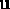is defined byThe BFGS formula is usually to be preferred GMW.

The step length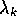in equation (4.24) is determined as for the Newton-Raphson method; i.e either by linear search, backtracking, or by deciding onand gradually changing it to unity as the solution is being approached. The algorithm for the BFGS update with backtracking is given in Algorithm 4.4.

The Secant method has become very popular for optimization: it converges fast (at best superlinear), it is stable, and spends relatively modest computing time at each iteration. The method is often being preferred over the Conjugate Gradients method, but the latter will have an advantage over the former for systems with large number of dimensions (e.g. 10,000). Since both the DFP and the BFGS updating formulas are guaranteed to uphold the positive definiteness of the initial approximation of the inverse Hessian (assuming that there are no roundoff errors that results in loss of positive definiteness), the Secant method will only be able to locate minimum points.Next: Orbits of Galaxies Up: Optimization Previous: The Newton-Raphson Method

Trond Hjorteland
Mon Jul 5 02:59:28 MET DST 1999